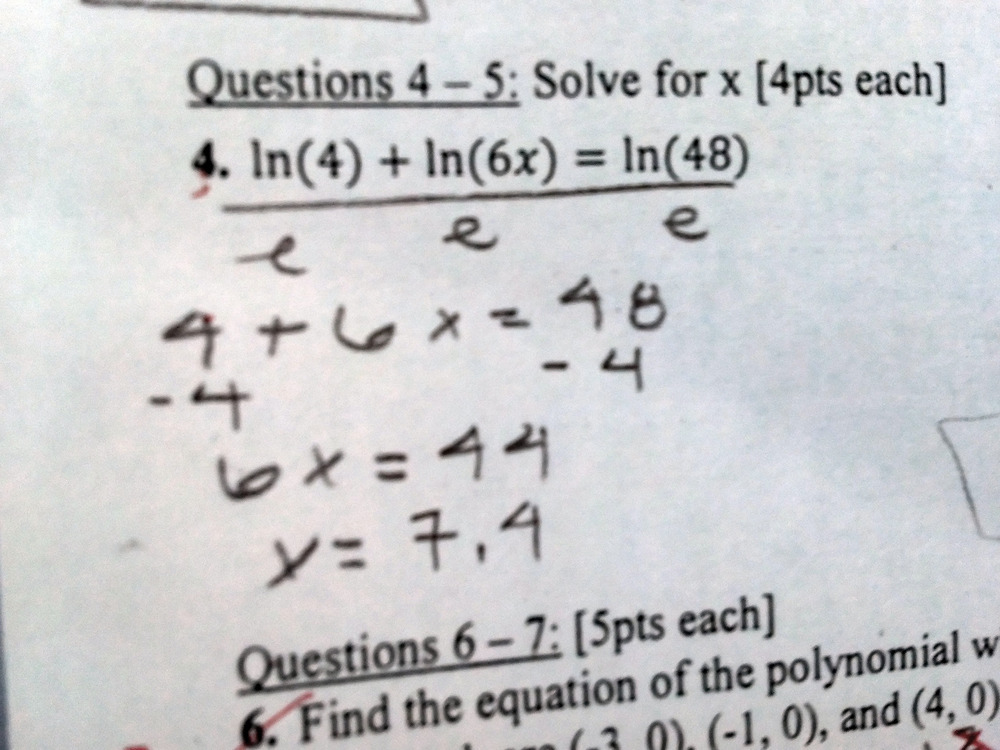Categories

## The Derivatives of e^x and ln(x)

What you need to know is that the student work is the stuff typed in red, and that this came on a take-home quiz:

Why does this student think that ln(e) = x^1. Or did I get that wrong? Are there ideas that “feel similar” that he’s confusing, or is it something else?

(Thanks Taylor!)

Categories

## ln(5) – ln(4) = ln

ln(5) – ln(4) = ln

Thanks, Jack!

Categories

## Constants and Exponents(Thanks to Pam for the submission.)

Categories

## Solving logarithmic equationsSelected by: Christopher Danielson

Question: What’s up with this? Why does this piece of student work matter? Does it?

Categories

## Dividing by “log”Last week we posted a somewhat similar mistake involving logarithms. Here’s the student work:While you should feel free to comment on today’s mistake independent of the earlier one, I think it would be especially productive to compare these two pieces of student work. Could the same student have done both pieces of work? Are there differences between the way each student understands logarithms?

By the way, we now support equation editing in LaTeX, so feel free to write things such as$\frac{2\log(6x)}{\log}$ in the comments. This post is also tagged with a Common Core Standard and categorized according the hierarchy that you see in the menu on the left.

Categories

## Dividing by “e”

Why did this student divide by “e”? What does that say about the way they think about logarithms? How would you help the student?